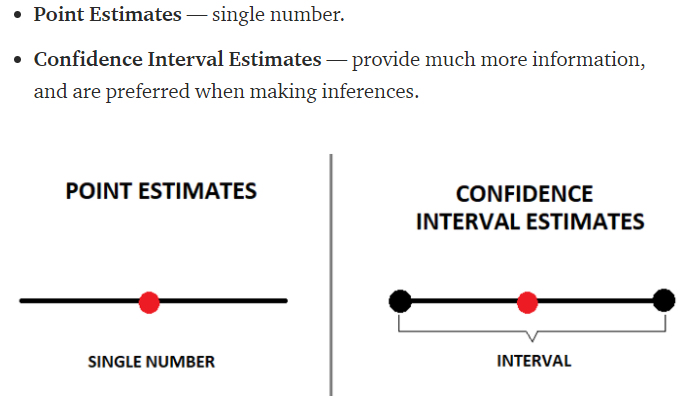# What is the difference between Point Estimates and Confidence Interval?

What is the difference between Point Estimates and Confidence Interval?

Based on a random sample of a population, a point estimate is the best estimate although it is not absolutely accurate. Furthermore, if you continuously retrieve random samples from the same population it is expected that the point estimate would vary from sample to sample.

On the other hand, a confidence interval is an estimate constructed on assumption that the true parameter will fall within a specified proportion regardeless of the number of samples analysed.A point estimate of a parameter is your estimate of a parameter value given some sort of algorithm (called a statistic). For example, the mean of n observations from a normal distribution is a statistic. Since the mean is also a Maximum Likelihood Estimate (MLE) of the true mean, you can say that the point estimate (the sample mean) is the best guess at the true mean given the logic of the MLE approach to estimation.

HOWEVER …

Is the point estimate actually equal to the true mean of the normal distribution? Almost certainly, the answer is No. All that we can say is that with probability (approximately) equal to 95%, the true mean lies in the interval

[Sample Mean - 2SE, Sample Mean + 2SE]

where SE is the standard error. The SE is a measure of accuracy. As the sample size n gets large, SE gets small. That is, the Sample Mean (the point estimate) gets closer and closer to the true mean as n increases.